# A student must make a buffer solution with a pH of 1.00, Determine which weak acid...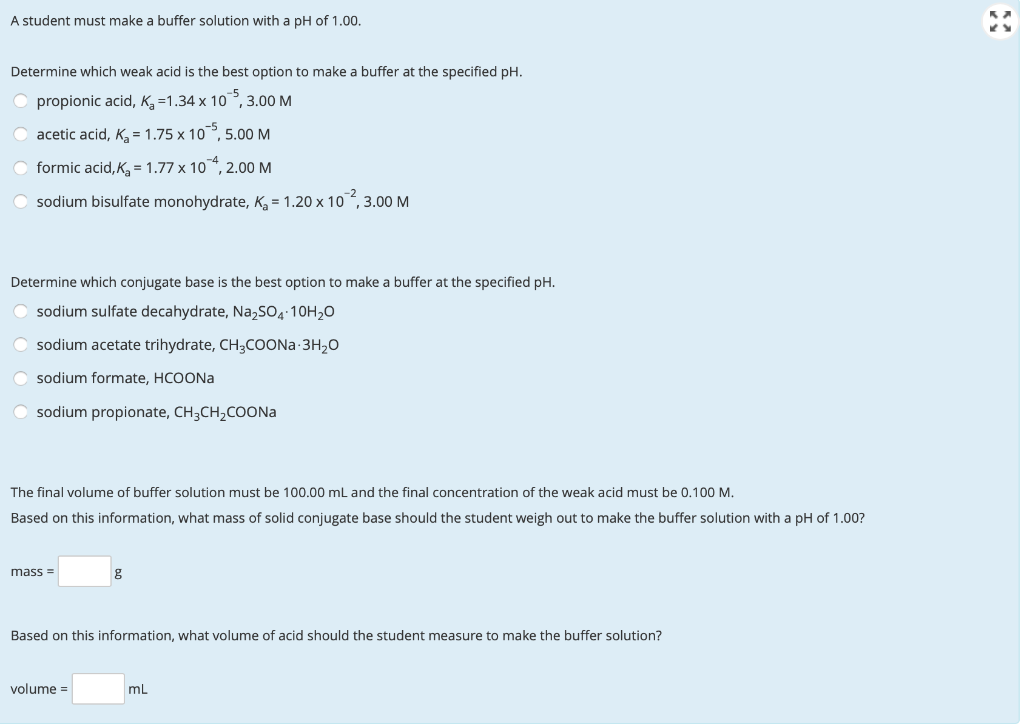A student must make a buffer solution with a pH of 1.00, Determine which weak acid is the best option to make a buffer at the specified pH. propionic acid, Kg =1.34 x 10,3.00 M acetic acid, Kg = 1.75 x 105,5.00 M formic acid, Kg = 1.77 x 104, 2.00 M sodium bisulfate monohydrate, K = 1.20 x 10,3.00 M Determine which conjugate base is the best option to make a buffer at the specified pH. sodium sulfate decahydrate, Na2SO4 10H20 sodium acetate trihydrate, CH3COONa 3H20 sodium formate, HCOONa sodium propionate, CH3CH2COONa The final volume of buffer solution must be 100.00 mL and the final concentration of the weak acid must be 0.100 M. Based on this information, what mass of solid conjugate base should the student weigh out to make the buffer solution with a pH of 1.00? mass = Based on this information, what volume of acid should the student measure to make the buffer solution? volume =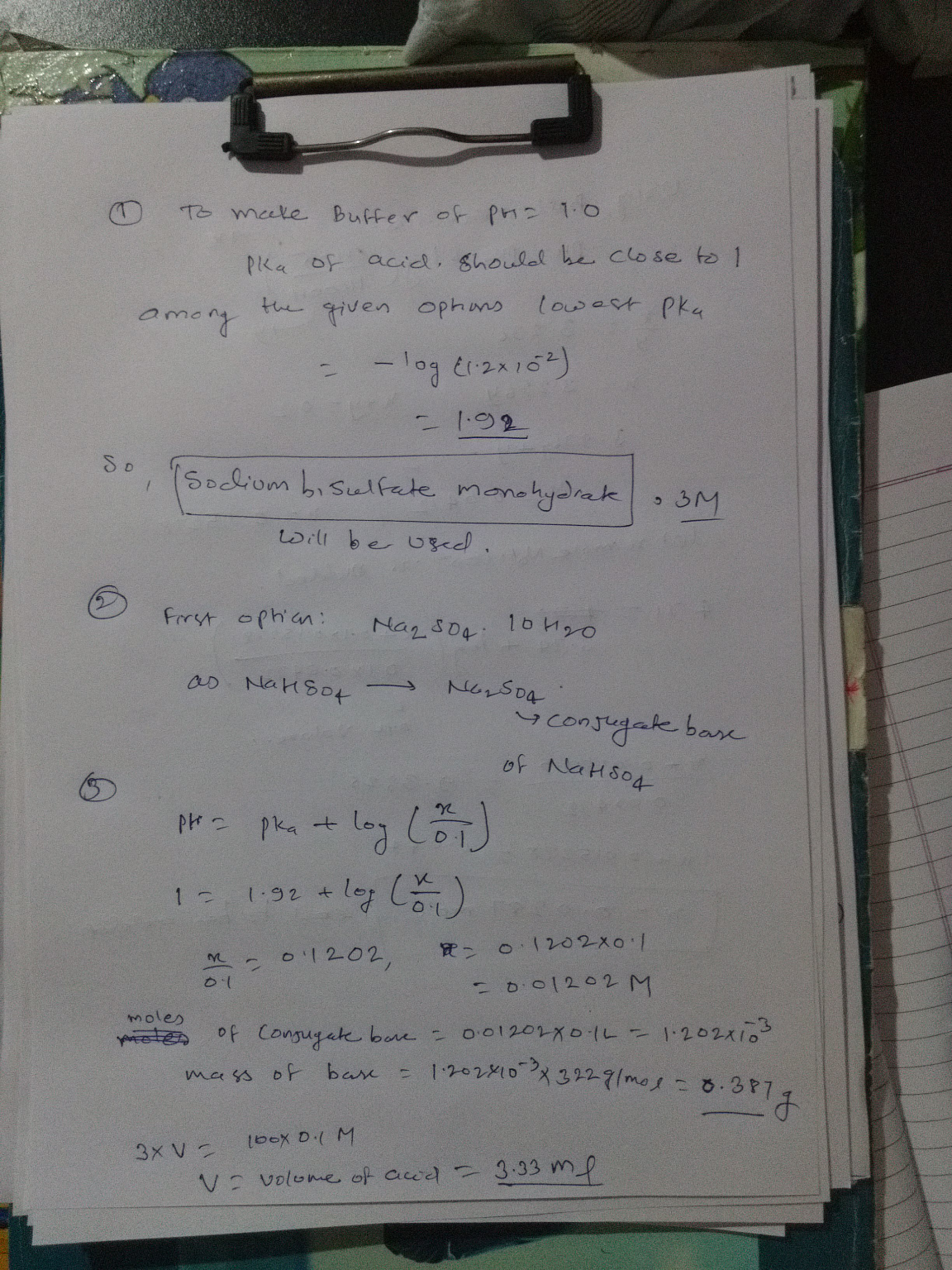#### Earn Coin

Coins can be redeemed for fabulous gifts.

Similar Homework Help Questions
• ### A student must make a buffer solution with a pH of 1.00. Determine which weak acid...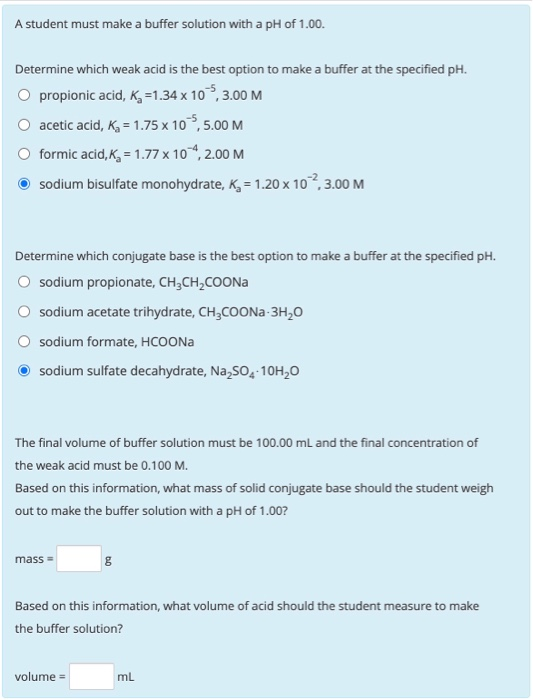A student must make a buffer solution with a pH of 1.00. Determine which weak acid is the best option to make a buffer at the specified pH. O propionic acid, K =1.34 x 10 5,3.00 M acetic acid, Ka = 1.75 x 10,5.00 M O formic acid, Kg = 1.77 x 104,2.00 M sodium bisulfate monohydrate, K = 1.20 x 102,3.00 M Determine which conjugate base is the best option to make a buffer at the specified pH. sodium...

• ### A student must make a buffer solution with a pH of 1.50. Determine which weak acid...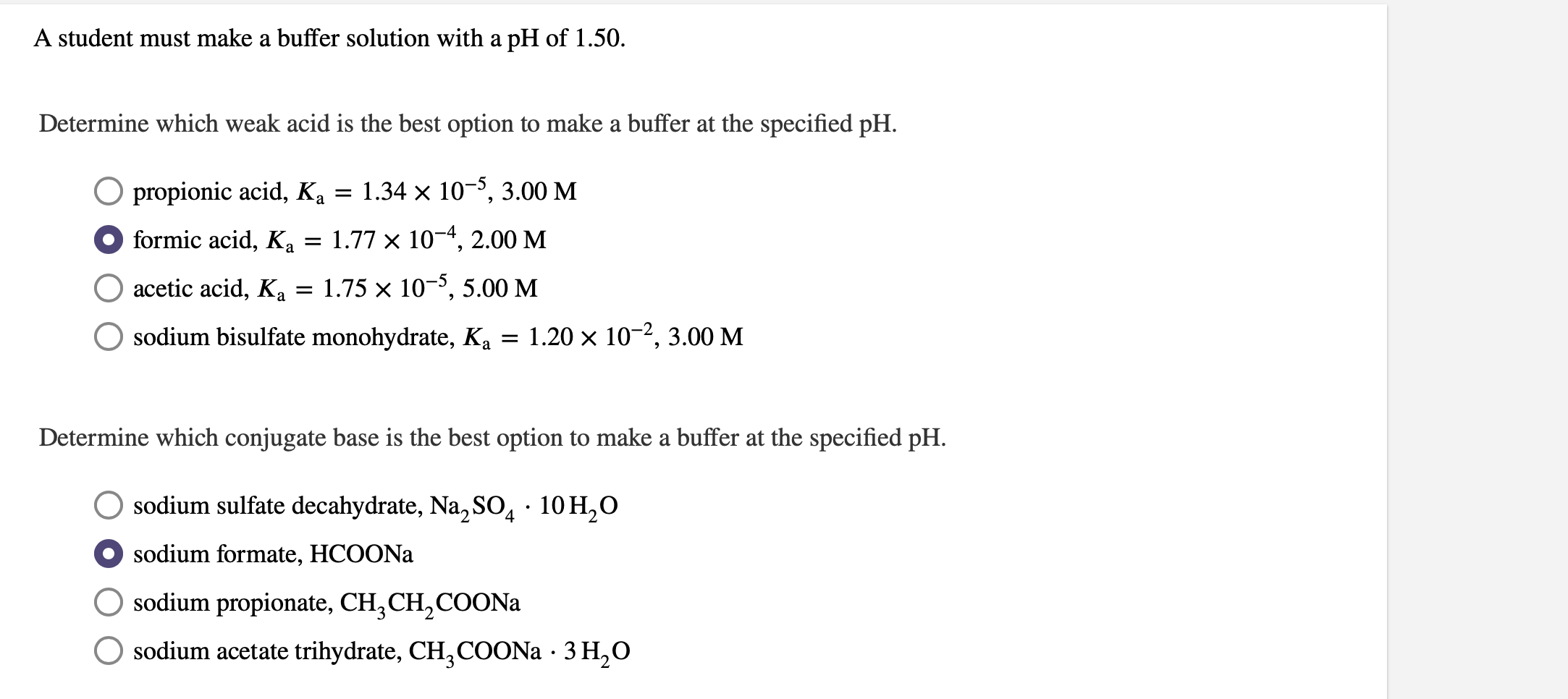A student must make a buffer solution with a pH of 1.50. Determine which weak acid is the best option to make a buffer at the specified pH. O propionic acid, Ka = 1.34 x 10-5, 3.00 M formic acid, Ka = 1.77 x 10-4, 2.00 M O acetic acid, Ka = 1.75 x 10-5,5.00 M O sodium bisulfate monohydrate, Ka = 1.20 x 10-2, 3.00 M Determine which conjugate base is the best option to make a buffer at...

• ### A student must make a buffer solution with a pH of 2.00. Determine which weak acid...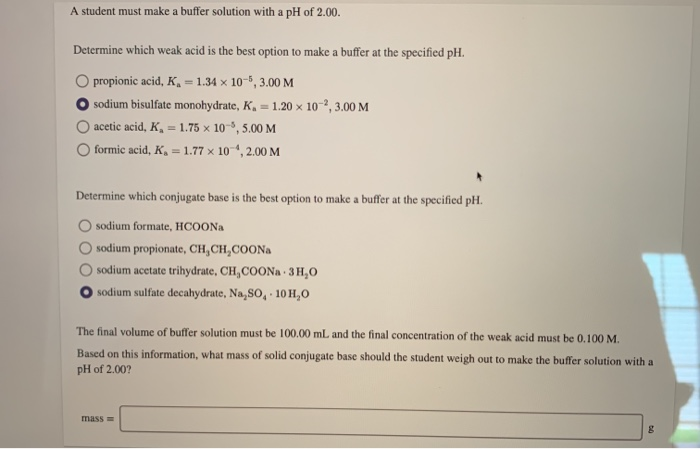A student must make a buffer solution with a pH of 2.00. Determine which weak acid is the best option to make a buffer at the specified pH. O propionic acid, K = 1.34 x 10-6, 3.00 M O sodium bisulfate monohydrate, K = 1.20 x 10,3.00 M acetic acid, K, = 1.75 x 10-6, 5.00 M formic acid, K, = 1.77 x 10 , 2.00 M Determine which conjugate base is the best option to make a buffer at...

• ### Determine which weak acid is the best option to make a buffer at the specified pH...

Determine which weak acid is the best option to make a buffer at the specified pH of 3.00. ***formic acid, ?a=1.77×10−4, 2.00 M propionic acid, ?a=1.34×10−5, 3.00 M phosphoric acid, ?a=7.52×10−3, 1.00 M acetic acid, ?a=1.75×10−5, 5.00 M Determine which conjugate base is the best option to make a buffer at the specified pH. sodium dihydrogen phosphate monohydrate, NaH2PO4⋅H2O ***sodium formate, HCOONa sodium acetate trihydrate, CH3COONa⋅3H2O sodium propionate, CH3CH2COONa The final volume of buffer solution must be 100.00 mL and...

• ### Consider how best to prepare one liter of a buffer solution with pH = 10.93 using one of the weak...

Consider how best to prepare one liter of a buffer solution with pH = 10.93 using one of the weak acid/conjugate base systems shown here. Weak Acid Conjugate Base Ka pKa HC2O4- C2O42- 6.4 x 10-5 4.19 H2PO4- HPO42- 6.2 x 10-8 7.21 HCO3- CO32- 4.8 x 10-11 10.32 How many grams of the sodium salt of the weak acid must be combined with how many grams of the sodium salt of its conjugate base, to produce1.00 L of a...

• ### The final volume of buffer solution must be 100.00 mL and the final concentration of the...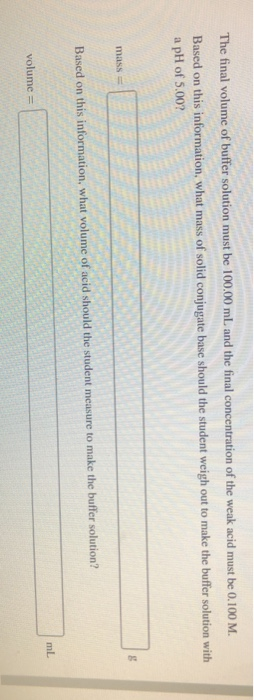The final volume of buffer solution must be 100.00 mL and the final concentration of the weak acid must be 0.100 M. Based on this information, what mass of solid conjugate base should the student weigh out to make the buffer solution with a pH of 5.00? MISS Based on this information, what volume of acid should the student measure to make the buffer solution? volume =

• ### 2. A Student wants to prepare 250.0 mL of pH 4.1 buffer solution. He is planning...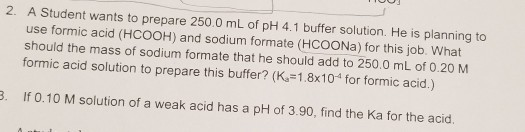2. A Student wants to prepare 250.0 mL of pH 4.1 buffer solution. He is planning to use formic acid (HCOOH) and sodium formate (HCOONa) for this job. What should the mass of sodium formate that he should add to 250,0 mL of 0.20 M formic acid solution to prepare this buffer? (K =1.8x10 for formic acid.) 3. If 0.10 M solution of a weak acid has a pH of 3.90, find the Ka for the acid.

• ### Consider how best to prepare one liter of a buffer solution with pH = 3.36 using...

Consider how best to prepare one liter of a buffer solution with pH = 3.36 using one of the weak acid/conjugate base systems shown here. Weak Acid Conjugate Base Ka pKa HC2O4- C2O42- 6.4 x 10-5 4.19 H2PO4- HPO42- 6.2 x 10-8 7.21 HCO3- CO32- 4.8 x 10-11 10.32 How many grams of the sodium salt of the weak acid must be combined with how many grams of the sodium salt of its conjugate base, to produce 1.00 L of...

• ### Calculate the amounts in grams of weak acid and conjugate base needed to make the buffer....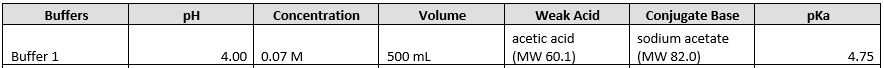Calculate the amounts in grams of weak acid and conjugate base needed to make the buffer. Buffers Concentration Volume Weak Acid Conjugate Base sodium acetate pH Ka acetic acid Buffer 1 500 mL 4.75

• ### pH and buffer solutions If a buffer solution is 0.150 M in a weak acid (K,...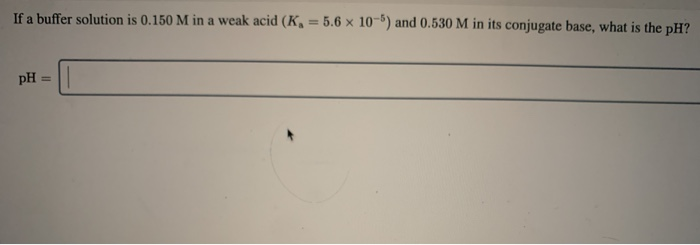pH and buffer solutions If a buffer solution is 0.150 M in a weak acid (K, = 5.6 x 10-) and 0.530 M in its conjugate base, what is the pH?